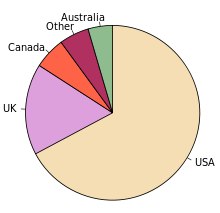# Univariate Function: Definition

A univariate function has only one variable.

Similarly, univariate equations, expressions, or polynomials only have one variable. Univariate analysis is a the simplest sort of data analysis, that only takes into account one variable or condition.

## Examples of Univariate Functions

One example of this type of function is:
f(x) = x2.
This function describes a change based on just one variable or condition, x. If x represents time and f(x) represents the number of political protesters in a city square, this equation might represent how the number of protesters changed over time.

Once you take into account other conditions, you make your function multivariate. For example, if we wanted to adjust for the amount of rain, and every mm of rain made 1 protester leave every minute, we might say
f(x) = x2 – 10xy.
This would no longer be a univariate function. It might be more accurate, but it would have lost much in simplicity.

## Describing Univariate Data

Functions or data in just one variable can be graphed with bar charts, histograms, frequency distribution tables, and pie charts.

We can also describe the patterns we find in them by giving the mean, mode, median, range, variance, and the standard deviation.

The image below is a pie chart showing the populations of English native speakers per country of residence.## Applications of Univariate Functions & Analysis

Functions and distributions of one variable are important because they give us a way to easily define what would be happening if we reduced the action on our target population to just one influencer. In clinical studies, analysis in one variable is always the first step in research. This type of analysis allows us to describe the variable distribution in a preliminary sample.

## References

Canova S, Cortinovis DL, Ambrogi F. How to describe univariate data. J Thorac Dis. 2017;9(6):1741–1743. doi:10.21037/jtd.2017.05.80. Retrieved from https://www.ncbi.nlm.nih.gov/pmc/articles/PMC5506131/ on August 17, 2019

CITE THIS AS:
Stephanie Glen. "Univariate Function: Definition" From StatisticsHowTo.com: Elementary Statistics for the rest of us! https://www.statisticshowto.com/univariate-function/